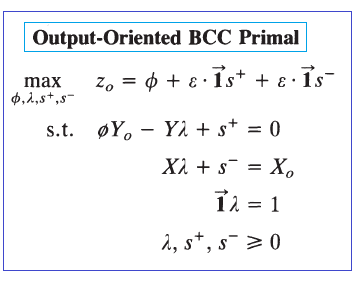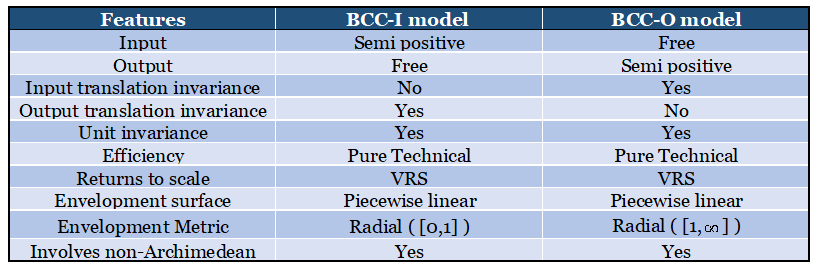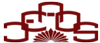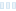# BASIC DEA MODELS- ENVELOPMENT FORMS <%=LablePriceTitle %>

CCR model

Charnes, Cooper and Rhodes (1978) first proposed DEA as an evaluation tool to measure and compare the relative efficiency of DMUs. Their model
(i) yields an objective evaluation of overall efficiency and
(ii) assumes constant returns to scale (CRS).

One version of a CCR model aims to minimize inputs while satisfying at least the given output levels. This is called the input-oriented model that is expressed as follows: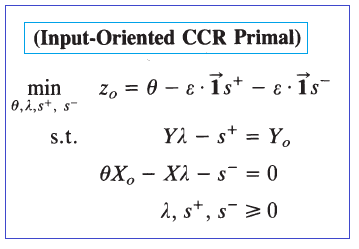There is another type of model called the output-oriented model that attempts to maximize outputs without requiring more of any of the observed input values that is expressed as follows: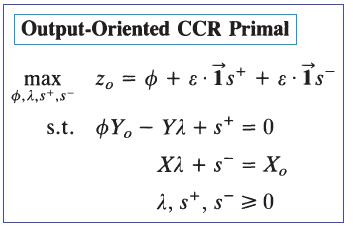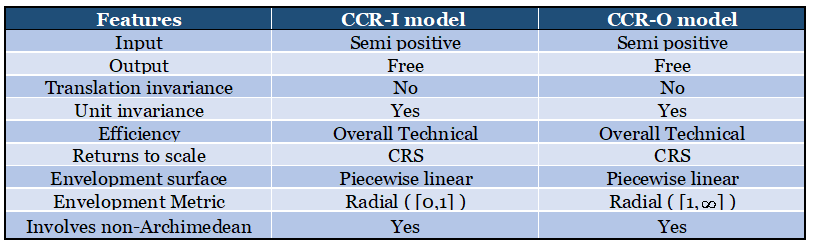BCC model

Banker, Charnes and Cooper (1984) extended the earlier work of Charnes et al. (1978) by providing for variable returns to scale (VRS). The BCC model (1984) distinguishes between technical and scale inefficiencies by
(i) estimating pure technical efficiency at the given scale of operation and
(ii) identifying whether increasing decreasing, or constant returns to scale possibilities are present for further exploitation.
The input-oriented VRS envelopment model is expressed as: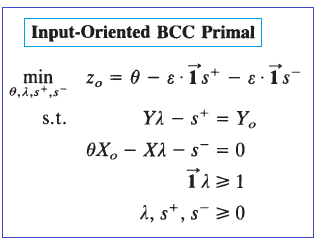The output-oriented VRS envelopment model is expressed as: